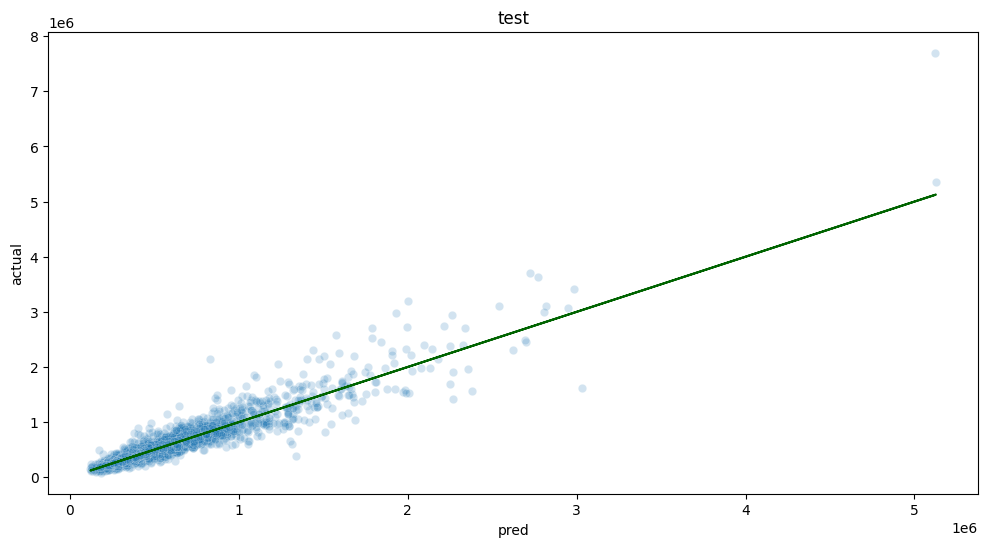## From Jupyter to Production

How to go from Jupyter Notebooks to Production Systems

The following tutorials are available from the Wallaroo Tutorials Repository.

# Stage 2: Training Process Automation Setup

Now that we have decided on the type and structure of the model from Stage 1: Data Exploration And Model Selection, this notebook modularizes the various steps of the process in a structure that is compatible with production and with Wallaroo.

We have pulled the preprocessing and postprocessing steps out of the training notebook into individual scripts that can also be used when the model is deployed.

Assuming no changes are made to the structure of the model, this notebook, or a script based on this notebook, can then be scheduled to run on a regular basis, to refresh the model with more recent training data. We’d expect to run this notebook in conjunction with the Stage 3 notebook, 03_deploy_model.ipynb. For clarity in this demo, we have split the training/upload task into two notebooks, 02_automated_training_process.ipynb and 03_deploy_model.ipynb.

## Resources

The following resources are used as part of this tutorial:

• data
• data/seattle_housing_col_description.txt: Describes the columns used as part data analysis.
• data/seattle_housing.csv: Sample data of the Seattle, Washington housing market between 2014 and 2015.
• code
• postprocess.py: Formats the data after inference by the model is complete.
• preprocess.py: Formats the incoming data for the model.
• simdb.py: A simulated database to demonstrate sending and receiving queries.
• wallaroo_client.py: Additional methods used with the Wallaroo instance to create workspaces, etc.

## Steps

The following steps are part of this process:

### Retrieve Training Data

Note that this connection is simulated to demonstrate how data would be retrieved from an existing data store. For training, we will use the data on all houses sold in this market with the last two years.

import numpy as np
import pandas as pd

import sklearn

import xgboost as xgb

import seaborn
import matplotlib
import matplotlib.pyplot as plt

import pickle

import simdb # module for the purpose of this demo to simulate pulling data from a database

from preprocess import create_features  # our custom preprocessing
from postprocess import postprocess    # our custom postprocessing

matplotlib.rcParams["figure.figsize"] = (12,6)

# ignoring warnings for demonstration
import warnings
warnings.filterwarnings('ignore')

conn = simdb.simulate_db_connection()
tablename = simdb.tablename

query = f"select * from {tablename} where date > DATE(DATE(), '-24 month') AND sale_price is not NULL"
print(query)

conn.close()
housing_data.loc[:, ["id", "date", "list_price", "bedrooms", "bathrooms", "sqft_living", "sqft_lot"]]

select * from house_listings where date > DATE(DATE(), '-24 month') AND sale_price is not NULL

id date list_price bedrooms bathrooms sqft_living sqft_lot
0 7129300520 2022-10-05 221900.0 3 1.00 1180 5650
1 6414100192 2022-12-01 538000.0 3 2.25 2570 7242
2 5631500400 2023-02-17 180000.0 2 1.00 770 10000
3 2487200875 2022-12-01 604000.0 4 3.00 1960 5000
4 1954400510 2023-02-10 510000.0 3 2.00 1680 8080
... ... ... ... ... ... ... ...
20518 263000018 2022-05-13 360000.0 3 2.50 1530 1131
20519 6600060120 2023-02-15 400000.0 4 2.50 2310 5813
20520 1523300141 2022-06-15 402101.0 2 0.75 1020 1350
20521 291310100 2023-01-08 400000.0 3 2.50 1600 2388
20522 1523300157 2022-10-07 325000.0 2 0.75 1020 1076

20523 rows × 7 columns

### Data transformations

To improve relative error performance, we will predict on log10 of the sale price.

Predict on log10 price to try to improve relative error performance

housing_data['logprice'] = np.log10(housing_data.list_price)

# split data into training and test
outcome = 'logprice'

runif = np.random.default_rng(2206222).uniform(0, 1, housing_data.shape)
gp = np.where(runif < 0.2, 'test', 'training')

hd_train = housing_data.loc[gp=='training', :].reset_index(drop=True, inplace=False)
hd_test = housing_data.loc[gp=='test', :].reset_index(drop=True, inplace=False)

# split the training into training and val for xgboost
runif = np.random.default_rng(123).uniform(0, 1, hd_train.shape)
xgb_gp = np.where(runif < 0.2, 'val', 'train')

# for xgboost
train_features = hd_train.loc[xgb_gp=='train', :].reset_index(drop=True, inplace=False)
train_features = np.array(create_features(train_features))
train_labels = np.array(hd_train.loc[xgb_gp=='train', outcome])

val_features = hd_train.loc[xgb_gp=='val', :].reset_index(drop=True, inplace=False)
val_features = np.array(create_features(val_features))
val_labels = np.array(hd_train.loc[xgb_gp=='val', outcome])

print(f'train_features: {train_features.shape}, train_labels: {len(train_labels)}')
print(f'val_features: {val_features.shape}, val_labels: {len(val_labels)}')

train_features: (13129, 18), train_labels: 13129
val_features: (3300, 18), val_labels: 3300


### Generate and Test the Model

Based on the experimentation and testing performed in Stage 1: Data Exploration And Model Selection, XGBoost was selected as the ML model and the variables for training were selected. The model will be generated and tested against sample data.


xgb_model = xgb.XGBRegressor(
objective = 'reg:squarederror',
max_depth=5,
base_score = np.mean(hd_train[outcome])
)

xgb_model.fit(
train_features,
train_labels,
eval_set=[(train_features, train_labels), (val_features, val_labels)],
verbose=False,
early_stopping_rounds=35
)

XGBRegressor(base_score=5.666446833601829, booster='gbtree', callbacks=None,
colsample_bylevel=1, colsample_bynode=1, colsample_bytree=1,
early_stopping_rounds=None, enable_categorical=False,
eval_metric=None, gamma=0, gpu_id=-1, grow_policy='depthwise',
importance_type=None, interaction_constraints='',
learning_rate=0.300000012, max_bin=256, max_cat_to_onehot=4,
max_delta_step=0, max_depth=5, max_leaves=0, min_child_weight=1,
missing=nan, monotone_constraints='()', n_estimators=100, n_jobs=0,
num_parallel_tree=1, predictor='auto', random_state=0, reg_alpha=0,
reg_lambda=1, ...)
In a Jupyter environment, please rerun this cell to show the HTML representation or trust the notebook.
XGBRegressor(base_score=5.666446833601829, booster='gbtree', callbacks=None,
         colsample_bylevel=1, colsample_bynode=1, colsample_bytree=1,
early_stopping_rounds=None, enable_categorical=False,
eval_metric=None, gamma=0, gpu_id=-1, grow_policy=&#x27;depthwise&#x27;,
importance_type=None, interaction_constraints=&#x27;&#x27;,
learning_rate=0.300000012, max_bin=256, max_cat_to_onehot=4,
max_delta_step=0, max_depth=5, max_leaves=0, min_child_weight=1,
missing=nan, monotone_constraints=&#x27;()&#x27;, n_estimators=100, n_jobs=0,
num_parallel_tree=1, predictor=&#x27;auto&#x27;, random_state=0, reg_alpha=0,
reg_lambda=1, ...)</pre>

print(xgb_model.best_score)
print(xgb_model.best_iteration)
print(xgb_model.best_ntree_limit)
0.07793614689092423
99
100

test_features = np.array(create_features(hd_test.copy()))
test_labels = np.array(hd_test.loc[:, outcome])

pframe = pd.DataFrame({
'pred' : postprocess(xgb_model.predict(test_features)),
'actual' : postprocess(test_labels)
})

ax = seaborn.scatterplot(
data=pframe,
x='pred',
y='actual',
alpha=0.2
)
matplotlib.pyplot.plot(pframe.pred, pframe.pred, color='DarkGreen')
matplotlib.pyplot.title("test")
plt.show()pframe['se'] = (pframe.pred - pframe.actual)**2

pframe['pct_err'] = 100*np.abs(pframe.pred - pframe.actual)/pframe.actual
pframe.describe()


pred
actual
se
pct_err

count
4.094000e+03
4.094000e+03
4.094000e+03
4094.000000

mean
5.340824e+05
5.396937e+05
1.657722e+10
12.857674

std
3.413714e+05
3.761666e+05
1.276017e+11
13.512028

min
1.216140e+05
8.200000e+04
1.000000e+00
0.000500

25%
3.167628e+05
3.200000e+05
3.245312e+08
4.252492

50%
4.568700e+05
4.500000e+05
1.602001e+09
9.101485

75%
6.310372e+05
6.355250e+05
6.575385e+09
17.041227

max
5.126706e+06
7.700000e+06
6.637466e+12
252.097895

rmse = np.sqrt(np.mean(pframe.se))
mape = np.mean(pframe.pct_err)

print(f'rmse = {rmse}, mape = {mape}')
rmse = 128752.54982046234, mape = 12.857674005250548

Convert the Model to Onnx
This step converts the model to onnx for easy import into Wallaroo.
import onnx
from onnxmltools.convert import convert_xgboost

from skl2onnx.common.data_types import FloatTensorType, DoubleTensorType

import preprocess

# set the number of columns
ncols = len(preprocess._vars)

# derive the opset value

from onnx.defs import onnx_opset_version
from onnxconverter_common.onnx_ex import DEFAULT_OPSET_NUMBER
TARGET_OPSET = min(DEFAULT_OPSET_NUMBER, onnx_opset_version())
# Convert the model to onnx

onnx_model_converted = convert_xgboost(xgb_model, 'tree-based classifier',
[('input', FloatTensorType([None, ncols]))],
target_opset=TARGET_OPSET)

# Save the model

onnx.save_model(onnx_model_converted, "housing_model_xgb.onnx")
With the model trained and ready, we can now go to Stage 3: Deploy the Model in Wallaroo.